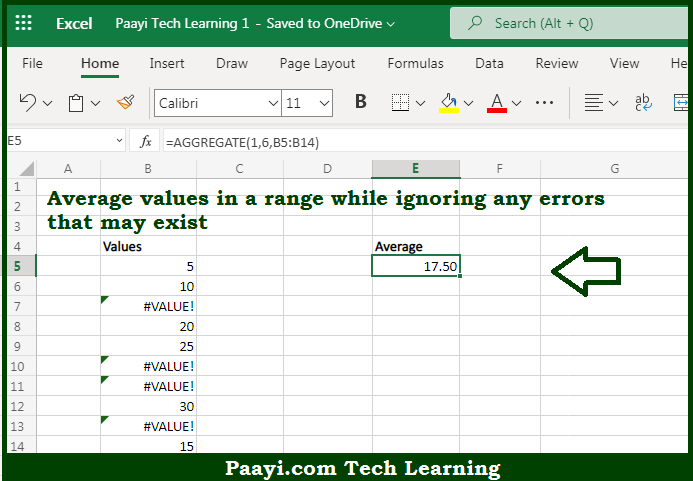# Learn How to AVERAGE and Ignore Errors in Microsoft Excel

Written by | 0 Comments | 582 Views

In this article, you will learn how to SUM various things in Microsoft Excel using a single/combination(s) of functions. You will also know how to AVERAGE and Ignore Errors and see the generic formula.

AVERAGE and Ignore Errors in Microsoft Excel

The main purpose of this formula is to average values in a range while ignoring any errors that may exist. Here we will learn how to average and ignore errors in a range in the workbook in Microsoft Excel. That implies, with the help of a formula based on the AVERAGEIF or AGGREGATE function you can able to average values in a range while ignoring any errors that may exist. So, with the help of this formula, you can able to average and ignore errors in a range in the workbook in Microsoft Excel.

General Formula to AVERAGE and Ignore Errors

=AGGREGATE(1,6,data)

The Explanation for the AVERAGE and Ignore ErrorsSo we know that with the help of the given formula above you can able to average values in a range while ignoring any errors that may exist. Here we will learn how to average and ignore errors in a range in the workbook in Microsoft Excel. As we know that the goal is to average a list of values that may contain errors. Usually, the AVERAGE function is used to calculate an average. However, if the data contains errors, the AVERAGE function will return an error. So, with the help of this formula, you can able to average and ignore errors in a range in the workbook in Microsoft Excel.